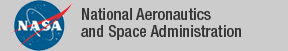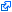### Top File of PVS vect_analysis Library

```
top: THEORY
%----------------------------------------------------------------------------
%
%    Vector Analysis Library
%
%    Some people will wonder why we did not exploit the more general notion of
%    continuity in the topology library.  The main reason is that the vectors
%    library would then have to import a huge number of theories.
%
%         Rick Butler        NASA Langley Research Center
%         Anthony Narkawicz  NASA Langley Research Center
%         Cesar Munoz        NASA Langley Research Center
%
%----------------------------------------------------------------------------
BEGIN

IMPORTING

% ----- generic theories ----------------------------------------------------

%    limit_real_vect,      % limit of functions over vectors
limit_vect_real,
limit_vect,
%    cont_real_vect,       % continuity of functions over vectors
%    cont_vect_real,
cont_vect,
metric_vect,
deriv_sigma,
deriv_dot_prod,
deriv_real_vect,
deriv_real_vect2,
deriv_cont_2D,
vect_metric_space,
vect3_metric_space,
vect3_Heine,

% ----- specific theories ----------------------------------------------------

limit_real_vect2,
%    limit_real_vect3,
limit_vect2_real,
limit_vect3_real,
limit_vect2_vect2,
cont_real_vect2,
%    cont_real_vect3,
cont_vect2_real,
cont_vect3_real,
cont_vect2_vect2,
vect2_cont_comp,
vect2_cont_comp2,      %% handles [T1 -> T2]
vect2_cont_dot,
vect_cont_2D,

vect_deriv_2D,
vect_chain_rule,
four_vects_2D_continuity,
vect2_metric_space,
vect2_Heine,
vect_vect_2D_continuity,

% ------- trig functions: continuity and derivatives (axiomatic versions of trig_fnd)

deriv_sincos_ax

END top

```
The tagidentifies links that are outside the NASA domain